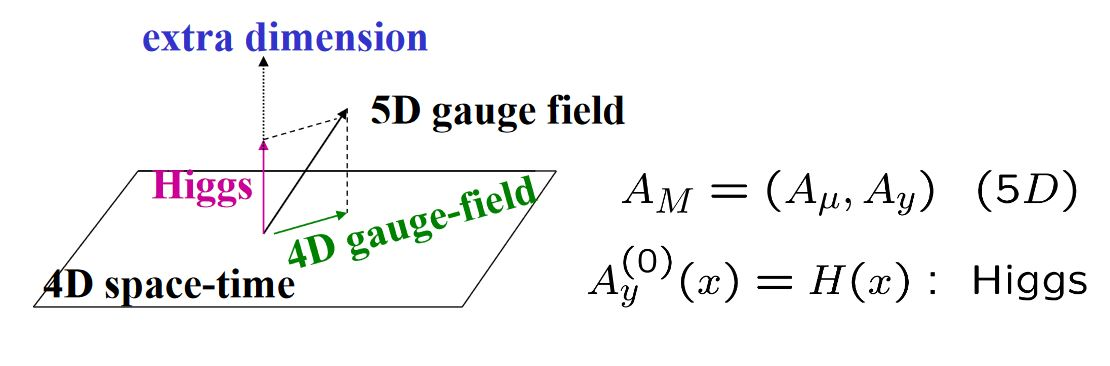nLab gauge-Higgs unification

Context

Fields and quanta

fields and particles in particle physics

and in the standard model of particle physics:

force field gauge bosons

scalar bosons

flavors of fundamental fermions in the
standard model of particle physics:
generation of fermions1st generation2nd generation3d generation
quarks ($q$)
up-typeup quark ($u$)charm quark ($c$)top quark ($t$)
down-typedown quark ($d$)strange quark ($s$)bottom quark ($b$)
leptons
chargedelectronmuontauon
neutralelectron neutrinomuon neutrinotau neutrino
bound states:
mesonslight mesons:
pion ($u d$)
ρ-meson ($u d$)
ω-meson ($u d$)
f1-meson
a1-meson
strange-mesons:
ϕ-meson ($s \bar s$),
kaon, K*-meson ($u s$, $d s$)
eta-meson ($u u + d d + s s$)

charmed heavy mesons:
D-meson ($u c$, $d c$, $s c$)
J/ψ-meson ($c \bar c$)
bottom heavy mesons:
B-meson ($q b$)
ϒ-meson ($b \bar b$)
baryonsnucleons:
proton $(u u d)$
neutron $(u d d)$

(also: antiparticles)

effective particles

hadrons (bound states of the above quarks)

solitons

in grand unified theory

minimally extended supersymmetric standard model

superpartners

bosinos:

dark matter candidates

Exotica

auxiliary fields

Contents

Idea

In particle physics, gauge-Higgs unification (Manton 79, Hosotani 83) also known as the Hosotani mechanism (see Hosotani 12) refers to hypothetical models of particle physics where the Higgs field on 4d spacetimes arises as a component of a gauge field in higher dimensions, either after Kaluza-Klein compactification or by passage to a 4d asymptotic boundary in a Randall-Sundrum model or similar.graphics grabbed from Lim 09

Gauge-Higgs unification is naturally combined with grand unification of gauge groups, whence one then also speaks of gauge-Higgs grand unification (e.g. Hosotani-Yamatsu 15). Also the Connes-Lott models realize gauge-Higgs unification, in a non-commutative geometric KK-compactification.

Attractive aspects of gauge-Higgs unification include the following:

1. a potential for providing a mechanism behind the Higgs-sector in the standard model of particle physics which could possibly solve the naturalness/hierarchy problem (e.g. Kaul 08, Csáki-Tanedo 13).

As such, gauge-Higgs unification is a possible alternative to MSSM-models.

2. a mechanism for ruling out proton decay in GUT-models, see below.

Properties

No proton-decay

Gauge-Higgs grand unification is claimed to predict stability of the proton, hence no proton decay (Hosotani-Yamatsu 15, Furui-Hosotani-Yamatsu 16, Sec. 2.6 Hosotani 17, Section 6).

General

The mechanism is due to

and was revived with:

• H. Hatanaka, T. Inami, C.S. Lim, The Gauge Hierarchy Problem and Higher Dimensional Gauge Theories, Mod. Phys. Lett. A13 (1998) 2601-2612 (arXiv:hep-th/9805067)

Review:

Deatiled phenomenology:

• Naoyuki Haba, Kazunori Takenaga, Toshifumi Yamashita, Higgs mass in the gauge-Higgs unification, Phys. Lett. B615 (2005) 247-256 (arXiv:hep-ph/0411250)

• C. S. Lim, Precision Tests and CP Violation in Gauge-Higgs Unification, 2009 (slides pdf)

• Jason Carson, Nobuchika Okada, 125 GeV Higgs boson mass from 5D gauge-Higgs unification, Progress of Theoretical and Experimental Physics, Volume 2018, Issue 3, March 2018, 033B03 (doi:10.1093/ptep/pty018)

Discussion in view of naturalness/hierarchy problem:

• Romesh K. Kaul, Naturalness and Electro-weak Symmetry Breaking (arXiv:0803.0381)

In $Spin(11)$-GUT (“$SO(11)$”):

Discussion in Spin(11)-GUT (“SO(11)-GUT”):

In $Spin(12)$-GUT (“$SO(12)$”):

Discussion in Spin(11)-GUT (“SO(11)-GUT”):

• S. Rajpoot and P. Sithikong, Implications of the $SO(12)$ gauge symmetry for grand unification, Phys. Rev. D 23, 1649 (1981) (doi:10.1103/PhysRevD.23.1649)

• Takaaki Nomura, Joe Sato, Standard(-like) Model from an $SO(12)$ Grand Unified Theory in six-dimensions with $S^2$ extra-space, Nucl.Phys.B811:109-122, 2009 (arXiv:0810.0898)

• Takaaki Nomura, Physics beyond the standard model with $S^2$ extra-space, 2009 (pdf, pdf)

• Cheng-Wei Chiang, Takaaki Nomura, Joe Sato, Gauge-Higgs unification models in six dimensions with $S^2/\mathbb{Z}_2$ extra space and GUT gauge symmetry (arXiv:1109.5835)